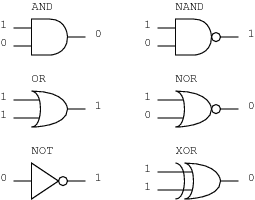# What is trivial and non-trivial gate in computing?

• Callaghan
In summary, a logic gate that takes a 0 as input and outputs a 1, or takes a 1 and outputs a 0 is called a trivial gate. Single input gates that take either zero or one as input and always output a zero or a one are also considered trivial.f

#### Callaghan

My question seems like it should be posted in Computer Engineering section, but below is how I found this word.

Quantum Computation And Quantum Information - by Michael A. Nielsen and Issac L. Chuang.
and I came across with the this word;

"1.3.1 Single qubit gates
Classical computer circuits consist of wires and logic gates. The wires are used to carry information around the circuit, while the logic gates perform manipulations of the information, converting it from one form to another. Consider, for example, classical single bit logic gates. The only non-trivial member of this class is the NOT gate, whose operation is defined by its truth table, in which 0 → 1 and 1 → 0, that is, the 0 and 1 states are
interchanged."

I was wondering the meaning of this word, and I googled, then one source came;
----------
logic gate (plural logic gates)

1. a physical device, typically electronic, which computes a Boolean logical output (0 or 1) from Boolean input or inputs according to the rules of some logical operator.
There are six non-trivial, symmetric, two-input, Boolean logic gates: AND, OR, XOR, NAND, NOR and XNOR.
----------
http://en.wiktionary.org/wiki/logic_gate

so,what is the meaning of trivial and non-trivial gate in "conventional" logic gate and what are the full examples?

Oh I see. They're talking about single input gates.

So, it can take one input (0 or 1), and provide one output (0 or 1).

A logic gate that is defined by the truth table (0>0, 1>1) is trivial because it does absolutely nothing. It might as well not be there at all.
So - of the two possible gates - the only non-trivial single bit gate is the NOT, which is defined as (0>1,1>0).

I'm confused.
trivial = single input?

wiktionary says
There are six non-trivial, symmetric, two-input, Boolean logic gates: AND, OR, XOR, NAND, NOR and XNOR.

but these logic gates mentioned above are not single input gates.

how do I distinguish that with

The only non-trivial member of this class is the NOT gate

?

trivial = does absolutely nothing?

I'm confused.
trivial = single input?
No. "Single input "means only one input signal (the gate is a back box that has a single "wire" going in). In the binary world that wire can only send a 0 or a 1 into the gate.

A gate that takes a 0 as input and outputs a 1, or takes a 1 and outputs a 0 could be useful in a circuit.

A gate that takes a 0 as input and outputs a 0, or takes a 1 and outputs a 1 accomplishes nothing - it might as well not be in the circuit at all.

There are six non-trivial, symmetric, two-input, Boolean logic gates: AND, OR, XOR, NAND, NOR and XNOR.
Yes, so for TWO-input gates (so the gate is a black box with two "wires" in), your input choices are: 00, 01,10,11
There are 6 ways inside the black box you could manipulate it so that the output is useful.

but these logic gates mentioned above are not single input gates.
No, they're two-input gates.

The only non-trivial member of this class is the NOT gate
That is referring to the single-input gates, as described at the top of this post.

trivial = does absolutely nothing?
I'd have said 'nothing useful', but yes. (I can imagine trivial gates that do something, just not a useful something. eg. a single-input gate could take either zero or one and always output a zero. They seem to consider that trivial if I read between the lines.)

Here's five two-input gates and one single-input gate (NOT).Last edited:
I see... Thank you very much.

Now I understand.